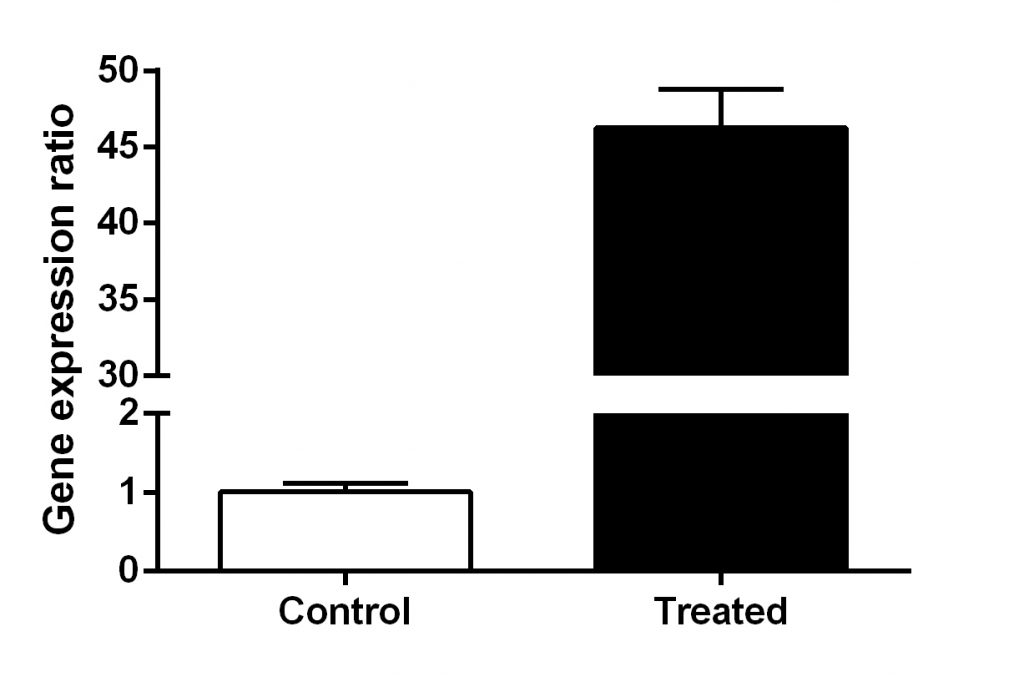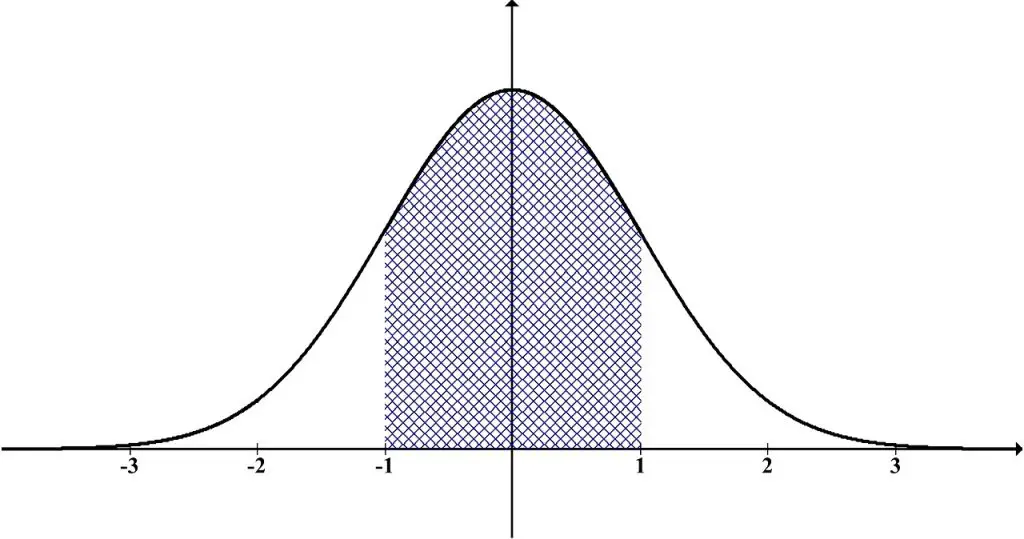# Independent (Unpaired) T-Test Assumptions

Before performing an independent (unpaired) t-test, there are five assumptions that need to be satisfied. Failure in meeting all of the assumptions can lead to inappropriate interpretations of the data.

Below is a list of assumptions which need to be met before performing an independent t-test.

## 1. The data is continuous

The data in question must be on a continuous scale. For example, measuring height in centimetres is a type of continuous dataset.

## 2. Only two groups are compared

For an independent t-test, only two groups should be present. For example, comparing a group of young and old individuals or males and females.An example of qPCR data comparing two independent groups

If there are more than two groups, you may require a One-Way ANOVA test. Conversely, if there is just a single group of data, it is likely a one-sample t-test is required.

## 3. The two groups should be independent

As the name of the test suggests, the two groups should be independent of each other. In other words, they are not related. For example, comparing heart rates between a group of exercisers and non-exercises are independent. They are not measured by the same individual.

If measurements from the same individuals are taken, before and after exercise for example, then this would be paired. A dependent (paired) t-test should be used in this instance.

## 4. The data should be normally distributed

Both datasets should be normally distributed. These should represent the classical Gaussian distribution or “bell-shaped curve” when plotted. Additionally, normality tests (e.g. Shapiro-Wilk) can be used to help determine this.An example of a “bell-shaped” Gaussian distribution

Check out our guides on normality testing in SPSS and GraphPad Prism.

## 5. The groups should have equal variance

The two groups should have the same variation in their data. In other words, they should have homogeneity of variance. If the standard deviations are similar, chances are they satisfy this assumption. Testing for equal variance can be done using Levene’s Test for Equality of Variances.

If the above assumptions have been confirmed, then an independent t-test can be performed.

Check out our guides for performing an independent t-test in: